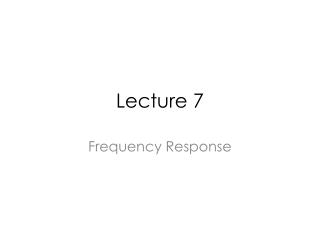DownloadDownload PresentationLecture 7

Lecture 7

Télécharger la présentationLecture 7

- - - - - - - - - - - - - - - - - - - - - - - - - - - E N D - - - - - - - - - - - - - - - - - - - - - - - - - - -
Presentation Transcript

1. Lecture 7 Frequency Response

2. Review of CS, CG and CD Amplifier

3. Voltage Gain of a CS Amplifier Interpretation: The resistance at the drain Divided by the resistance in the source path

4. Voltage Gain of a CD Amplifier

5. Voltage Gain of a CG Amplifier If RS=0 and channel length modulation is ignored, Av is

6. Resistance into the Drain Terminal

7. Resistance into the Source Terminal

8. Miller Effect

9. Miller’s Theorem

10. Typical Application of Miller’s Theorem Miller’s theorem is useful when Z appears in parallel with the main signal (i.e. the amplifier)

11. Limitation of Miller’s Theorem Limitations: Interaction of poles through R3 and C3.

12. Association of Poles with Nodes Each pole is determined by the product of Total capacitance seen from each node to ground Total resistance seen at the node to ground “Each node in the circuit contributes one pole to the transfer function”

13. Common-Gate Example

14. CS Stage • Output Impedance • Input Impedance • “Nodal Method” • Miller Approximation • “Zx” method • Equivalent Circuit Analysis • KCL • Dominant pole

15. High Frequency Model of CS Stage

16. CS Trade-Off For Same IOUT, L↓→W↓→GDS↑(Ro↓) →CDS ↓ Specs: AV=10 Vo,cm=0.6V I(M1)=10 uA gm=AV/RD Gmoverid_1=16.67 Trade-offs in GDS and parasitic capacitance.

17. CS Trade-Off For Same IOUT, L↓→W↓→GDS↑(Ro↓) →CDS ↓ Specs: Vo,cm=0.6V gm=AV/RD Difficult to achieve high gain and high speed at the same time!

18. Output Impedance Only Valid if Rs is large!

19. Input Impedance High frequency approximation Exclude CGS (First order model)

20. Input Impedance (KCL) High frequency approximation Exclude CGS (In parallel with CGS)

21. “Nodal Method”(Miller Approximation) It is important to identify the high impedance node! Numerical example: RS=50 Ohms L=2.0 um AV=15 fin=4.65 GHz fout=69.9 MHz 16(10.40fF) 517.8 fF CDB=27.51 fF, RD=60 KOhm

22. Transfer Function

23. “Nodal Method”(Refined Miller Approximation) (If RS is large!) (Capacitive) (Resistive)

24. Equivalent Circuit Analysis

25. Comparison to Miller Approximation

26. Dominant Pole Approximation

27. Transmission Zero Transmission Zero Finding a transmission zero in effective Gm.

28. Source Follower (Strong interaction between XY, making it difficult to associate each pole with each node)

29. Source Follower

30. Transmission Zero

31. Input Impedance

32. Analysis of Input Impedance Miller Approximation: Av: (Negative Resistance) Can be used to oscillators.

33. Output Impedance

34. Equivalent Output Impedance

35. Issues

36. Common Gate

37. Cascode (Gain from A to X)

38. DC Input Resistance Will a large Rin increase the miller effect of CS dramatically?

39. Input Resistance of Common Gate Note that ZL is not infinity if RD is replaced by a current source because ZL is in parallel with CD.

40. Differential Pair (Differential Mode) (Differential Half Circuit)

41. Differential Pair (Common-Mode) W3 is made as large as possible to minimize VDSAT.

42. Consequence of Limited CMRR

43. Differential Pair with High Impedance Load AC Ground (Dominant Pole)

44. Differential Pair Example GM=166.19 uS GDS=1.3552 uS RD=90 Kohm

45. AC analysis

46. Use the Waveform Calculator Add voltages to the calculator Press Evalbefore you plot

47. Plot in Magnitude/dB

48. Transfer Function 3dB Bandwidth: 317.629 MHz

49. Differential Pair with Current Mirror

50. Small Signal Equivalent Model (Transmission Zero)